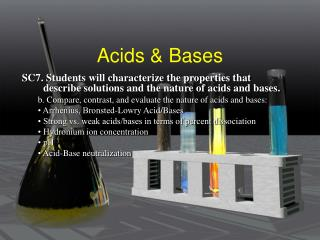DownloadDownload PresentationAcids & Bases

# Acids & Bases

Download Presentation## Acids & Bases

- - - - - - - - - - - - - - - - - - - - - - - - - - - E N D - - - - - - - - - - - - - - - - - - - - - - - - - - -
##### Presentation Transcript

1. Acids & Bases SC7. Students will characterize the properties that describe solutions and the nature of acids and bases. b. Compare, contrast, and evaluate the nature of acids and bases: • Arrhenius, Bronsted-Lowry Acid/Bases • Strong vs. weak acids/bases in terms of percent dissociation • Hydronium ion concentration • pH • Acid-Base neutralization

2. Properties of Acids: Sour Taste Electrolytes Change indicator colors React with metals to form hydrogen React with carbonates to form carbon dioxide React with bases to form salt & water Properties of bases: Bitter Feel Slippery Electrolytes Change indicator colors React with acids to produce salt & water Properties

3. What Are Acids? • Definitions • Arrhenius acid: Produce H+ ion • Arrhenius base: Produce OH- ion • Bronsted-Lowery Acid: H+ donor • Bronsted Lowery Base: H+ acceptor • **More Broad definition than Arrhenius** • Lewis acid- can accept pr of e- to form a covalent bond (H+) • Lewis base- can donate pr of e- to form a covalent bond (OH-) • Look for lone pr • ** is more broad than other two def Amphoteric- can be acid or base

4. Arrhenius acid • Acids Form H+ Ions when Dissolved in Water Ex HCl + H20 dH+ + Cl- + H20 Actually it forms H3O+ & Cl-

5. HCl +H2O  H3O+ + Cl- Monoprotic Acid- Produces 1 Hydrogen Ion Hydrogen Ion concentration can be measured in molarity H2SO4+2H2O  2H3O++ SO4-2 Di-protic acids- Produces 2 Hydrogen Ions

6. Hydronium IonConcentration • H+ or H3O ion concentration can be measured in molarity • Depends on # of Hydrogen Ions per Mole of Acid. • Monoprotic 1x HCl • Diprotic 2x H2SO4 • Triprotic 3x H3PO4 More on That Later?

7. Arrhenius Base • Form a Hydroxide Ion (OH-) During Solvation • NaOH + H2O d Na+ + OH- + H2O • Name Bases according to Ionic Rules • Most are hydroxides • NaOH – sodium hydroxide • OH- Ion Concentration can also be measured in Molarity

8. Neutralization • - Double displacement Reaction • Acid + Base  salt + water • Nitric acid & calcium hydroxide • Sulfuric acid & aluminum hydroxide • Can find acid & base that form salt • NaCl • NaHCO3

9. Self Ionization Of Water • H2O (HOH) can self Ionize into a H+ & an OH- • H2O + H2O  H3O+ + OH- • Hydronium ion H3O+ • Hydroxide ion OH- • Only 1X 10 –14 M of water molecules do this so [H3O+][OH-] = 1X 10 –14 M • If [H3O+]=[OH-] neutral solution • If [H3O+]>[OH-] acidic solution • If [H3O+]<[OH-] basic or alkaline solution

10. Ionization Constants • A constant (k), is a quantitative measure of the strength of an acid in solution. It is the equilibrium constant for a chemical reaction known as dissociation • HA  H+ + A-

11. Strengths of Acids and Bases • The strength of an acid or a base, is a measure of how many ions are formed when dissolved in water • Strong Acids Disassociate completely • Weak Acids do not completely Disassociate • Weak acids have Ka

12. Acids HCl HBr HI HNO3 H2SO4 HClO3 HClO4 Bases LiOH NaOH KOH RbOH CsOH Ca(OH)2 Sr(OH)2 Mg(OH)2 Strong Acids & Bases

13. pH Scale • pH=-Log[H3O] • pOH=-log[OH-] • pH+pOH=14 • pH 1-6 7 8-14 • Acid neutral base • pOH 14-8 7 6-1 • pH +pOH=14 • Use to find one given the other. • Find the pOH if the pH is 8.2.

14. Calculate the pH of a solution of HCl if the [H3O+] is 0.234 M 1) A 0.001 M solution of HCl 2) A 0.09 M solution of HBr 3) A 1.34 x 10-4 M solution of HCl 4) A 2.234 x 10-6 M solution of HI 5) A 7.98 x 10-2 M solution of HNO3